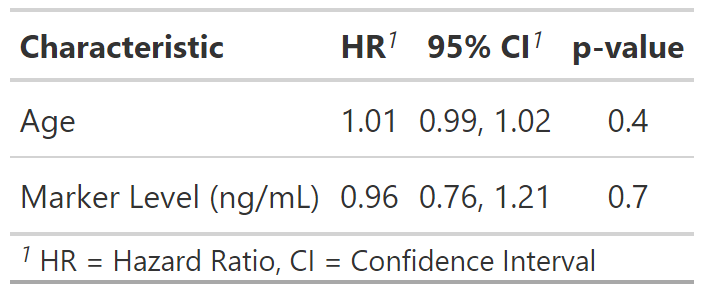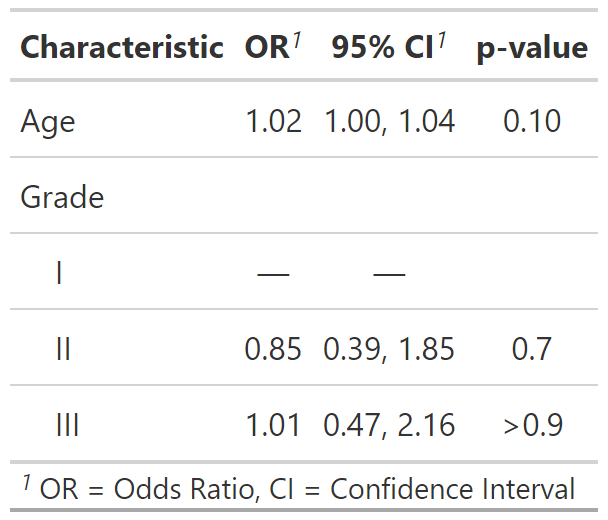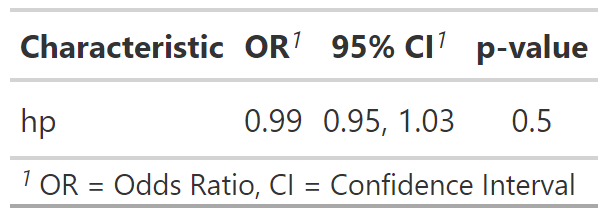This function takes a regression model object and returns a formatted table that is publication-ready. The function is highly customizable allowing the user to obtain a bespoke summary table of the regression model results. Review the tbl_regression vignette for detailed examples.

tbl_regression(x, ...)

# S3 method for default
tbl_regression(
x,
label = NULL,
exponentiate = FALSE,
include = everything(),
show_single_row = NULL,
conf.level = NULL,
intercept = FALSE,
estimate_fun = NULL,
pvalue_fun = NULL,
tidy_fun = NULL,
conf.int = NULL,
...
)

## Arguments

x

Regression model object

...

Not used

label

List of formulas specifying variables labels, e.g. list(age ~ "Age", stage ~ "Path T Stage")

exponentiate

Logical indicating whether to exponentiate the coefficient estimates. Default is FALSE.

include

Variables to include in output. Input may be a vector of quoted variable names, unquoted variable names, or tidyselect select helper functions. Default is everything().

show_single_row

By default categorical variables are printed on multiple rows. If a variable is dichotomous (e.g. Yes/No) and you wish to print the regression coefficient on a single row, include the variable name(s) here--quoted and unquoted variable name accepted.

conf.level

Must be strictly greater than 0 and less than 1. Defaults to 0.95, which corresponds to a 95 percent confidence interval.

intercept

Logical argument indicating whether to include the intercept in the output. Default is FALSE

estimate_fun

Function to round and format coefficient estimates. Default is style_sigfig when the coefficients are not transformed, and style_ratio when the coefficients have been exponentiated.

pvalue_fun

Function to round and format p-values. Default is style_pvalue. The function must have a numeric vector input (the numeric, exact p-value), and return a string that is the rounded/formatted p-value (e.g. pvalue_fun = function(x) style_pvalue(x, digits = 2) or equivalently, purrr::partial(style_pvalue, digits = 2)).

tidy_fun

Option to specify a particular tidier function for the model. Default is to use broom::tidy, but if an error occurs then tidying of the model is attempted with parameters::model_parameters(), if installed.

add a reference value. Default is FALSE

conf.int

Logical indicating whether or not to include a confidence interval in the output. Defaults to TRUE.

## Value

A tbl_regression object

## Methods

The default method for tbl_regression() model summary uses broom::tidy(x) to perform the initial tidying of the model object. There are, however, a few models that use modifications.

• "parsnip/workflows": If the model was prepared using parsnip/workflows, the original model fit is extracted and the original x= argument is replaced with the model fit. This will typically go unnoticed; however,if you've provided a custom tidier in tidy_fun= the tidier will be applied to the model fit object and not the parsnip/workflows object.

• "survreg": The scale parameter is removed, broom::tidy(x) %>% dplyr::filter(term != "Log(scale)")

• "multinom": This multinomial outcome is complex, with one line per covariate per outcome (less the reference group)

• "gam": Uses the internal tidier tidy_gam() to print both parametric and smooth terms.

• "tidycrr": Uses the tidier tidycmprsk::tidy() to print the model terms.

• "lmerMod", "glmerMod", "glmmTMB", "glmmadmb", "stanreg", "brmsfit": These mixed effects models use broom.mixed::tidy(x, effects = "fixed"). Specify tidy_fun = broom.mixed::tidy to print the random components.

## Example Output

Example 1Example 2Example 3See tbl_regression vignette for detailed examples

Review list, formula, and selector syntax used throughout gtsummary

Other tbl_regression tools: add_global_p(), add_q(), bold_italicize_labels_levels, combine_terms(), inline_text.tbl_regression(), modify, tbl_merge(), tbl_split(), tbl_stack(), tbl_strata()

## Author

Daniel D. Sjoberg

## Examples

# \donttest{
# Example 1 ----------------------------------
library(survival)
tbl_regression_ex1 <-
coxph(Surv(ttdeath, death) ~ age + marker, trial) %>%
tbl_regression(exponentiate = TRUE)

# Example 2 ----------------------------------
tbl_regression_ex2 <-
glm(response ~ age + grade, trial, family = binomial(link = "logit")) %>%
tbl_regression(exponentiate = TRUE)

# Example 3 ----------------------------------
suppressMessages(library(lme4))
tbl_regression_ex3 <-
glmer(am ~ hp + (1 | gear), mtcars, family = binomial) %>%
tbl_regression(exponentiate = TRUE)
# }